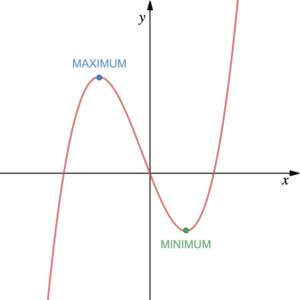# Stationary Points (turning points) – maxima & minima

## What are Stationary Points?Stationary points (or turning/critical points) are the points on a curve where the gradient is 0. This means that at these points the curve is flat. Usually, the gradient of a curve is always changing and so the gradient is only 0 instantaneously (unless the curve is a flat line, in which case, the gradient is always 0).

A MAXIMUM is located at the top of a peak on a curve. Conversely, a MINIMUM if it is at the bottom of a trough.

A stationary point can be found by solving $\frac{dy}{dx}=0$, i.e. finding the x coordinate where the gradient is 0. See more on differentiating to find out how to find a derivative.

See Example 1.

Click here for an online tool for checking your stationary points. Page 21 onwards of the StudyWell Differentiation eGuide has more on Stationary Points including exam-style questions.

## Classifying Stationary Points

For certain functions, it is possible to differentiate twice (or even more) and find the second derivative. It is often denoted as $f”(x)$ or $\frac{d^2y}{dx^2}$. For example, given that $f(x)=x^7-x^5$ then the derivative is $f'(x)=7x^6-5x^4$ and the second derivative is given by $f”(x)=42x^5-20x^3$.

The second derivative can tell us something about the nature of a stationary point:

• For a MINIMUM, the gradient changes from negative to 0 to positive, i.e. the gradient is increasing. Hence, the second derivative is positive – $f”(x)> 0$.
• For a MAXIMUM, the gradient changes from positive to 0 to negative, i.e. the gradient is decreasing. Hence, the second derivative is negative – $f”(x)< 0$.

We can classify whether a point is a minimum or maximum by determining whether the second derivative is positive or negative. This is done by putting the $x$-coordinates of the stationary points into $f”(x)$.

Page 21 onwards of the StudyWell Differentiation eGuide has more on Stationary Points including exam-style questions.

## Examples

Find the stationary points on the curve $y=\frac{2}{3}x^3-5x^2+8x-4$.

Find and classify the stationary points of $f(x)=x^3-2x^2+x-5$.